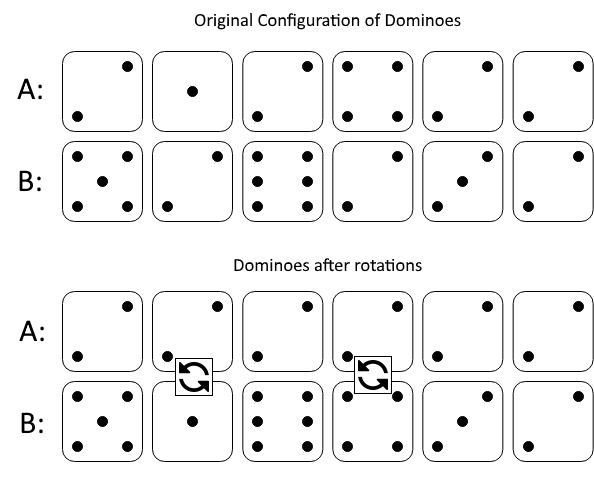# 行相等的最少多米诺旋转

## 1007. 行相等的最少多米诺旋转 (Medium)```输入：A = [2,1,2,4,2,2], B = [5,2,6,2,3,2]

```

```输入：A = [3,5,1,2,3], B = [3,6,3,3,4]

```

1. `1 <= A[i], B[i] <= 6`
2. `2 <= A.length == B.length <= 20000`

[贪心算法] [数组]